Example Questions

Example Question #1 : Monomials

Mike wants to sell candy bars for a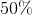profit. If he sells each bar for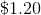, how much did each bar cost him?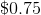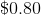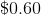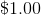Explanation:

In order to solve this problem, set up the following equation: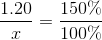Cross multiply: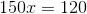Divide: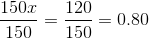The original cost of the of each candy bar isExample Question #2 : Monomials

Choose the answer that is the simplest form of the following expression of monomial quotients: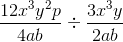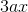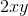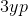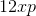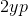Explanation:To divide monomial quotients, simply invert the divisor and multiply: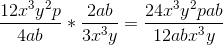Then, reduce: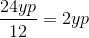Example Question #1 : How To Divide Monomial Quotients

Choose the answer that is the simplest form of the following expression of monomial quotients: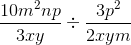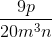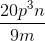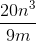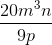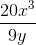Explanation:To find your answer, you have to invert the divisor and multiply across: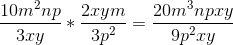Then, reduce:Example Question #4 : Monomials

Multiply: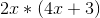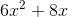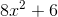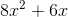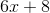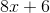Explanation:

To solve you must multiplyby both terms in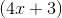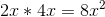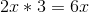Example Question #5 : Monomials

Multiply: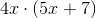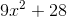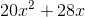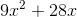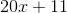Explanation:

Multiply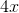by both terms in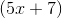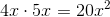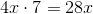Example Question #6 : Monomials

Multiply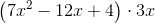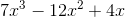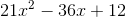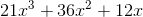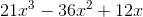Explanation:

When multiplying a polynomial by a monomial, each term in the polynomial gets multiplied by the monomial. Calculate each term one at a time, then add the results to get the final answer. In this case, we start by multiplying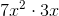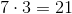and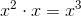, thus we get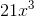. For the second term of the polynomial, we multiply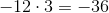and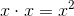, resulting in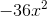. Finally, we multiply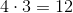and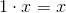, resulting in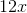. Adding the three terms that we just found, we come to the answer of.

Example Question #7 : Monomials

Choose the answer that is the best solution to the following expression of monomial quotients: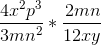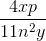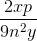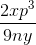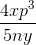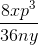Explanation:To multiply monomial quotients, treat them as you would any other fraction. Combine like terms wherever possible: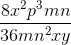Then, you need to reduce:Example Question #8 : Monomials

Choose the answer that is the simplest form of the following expression of monomial quotients: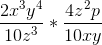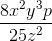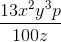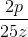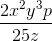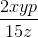Explanation:To simplify, first multiply across: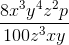Then, reduce: